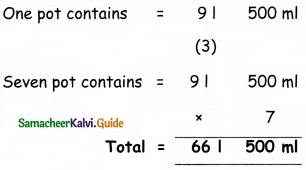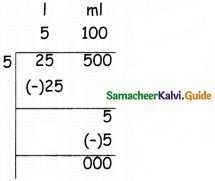Students can download 5th Maths Term 2 Chapter 4 Measurements Ex 4.2 Questions and Answers, Notes, Samacheer Kalvi 5th Maths Guide Pdf helps you to revise the complete Tamilnadu State Board New Syllabus, helps students complete homework assignments and to score high marks in board exams.

## Tamilnadu Samacheer Kalvi 5th Maths Solutions Term 2 Chapter 4 Measurements Ex 4.2

Question 1.
Fill in the blanks
(i) ________ is the smallest metric measure of capacity.
Milliliter

(ii) _______ is the largest unit of volume and equals ________ litres
Kilometer, 1000(iii) 7 kI 301 = _____l.
7030

(iv) 5 l 400 ml = ____ ml.
5400

(v) 1300ml = ____ l ____ ml
1, 300Question 2.
Match the following

 1. 4500 ml 6 l 500 ml 2. 3250 ml 8 l 200 ml 3. 6500 ml 7 l 50 ml 4. 8200 ml 4 l 500 ml 5. 7050 ml 3 l 250 ml

 1. 4500 ml 4 l 500 ml 2. 3250 ml 3 l 250 ml 3. 6500 ml 6 l 500 ml 4. 8200 ml 8 l 200 ml 5. 7050 ml 7 l 50 mlQuestion 3.
(i) 400 l; 50 l; 500 ml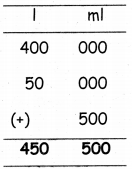sum = 450 l 500 ml

(ii) 3 kl; 400 I; 3 ml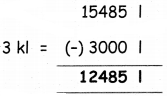sum = 3400 l 3 ml

(iii) 1400 ml; 5680 ml; 280 l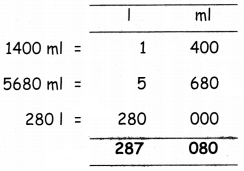sum = 287 l 080 mlQuestion 4.
Subtract:
(i) 3 kl from 15485 lDifference = 12485 l

(ii) 15 kl from 20 kl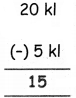Difference = 15 kl

(iii) 345 ml from 5 l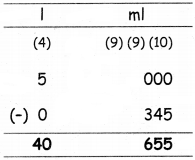Difference = 5 l 655 mlQuestion 5.
Multiply the following:
(i) 31200 ml × 8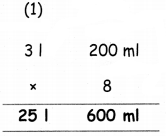Product = 25 l 600 ml

(ii) 4 I 450 ml × 4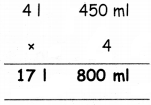Product = 17 l 800 ml

(iii) 51300 ml × 5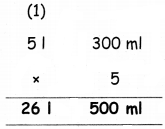Product = 26 l 500 ml(iv) 61700 ml × 6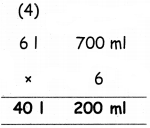Product = 40 l 200 ml

Question 6.
Divide the following:
(i) 18 l 240 ml ÷ 6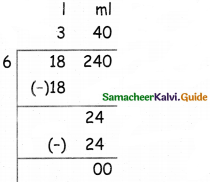18 l 240 ml ÷ 6 = 3 l 40 ml

(ii) 20 l 600 ml ÷ 2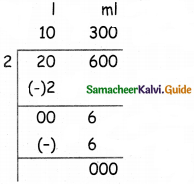20 l 600 ml ÷ 2 = 10 l 300 ml(iii) 21 l 490 ml ÷ 7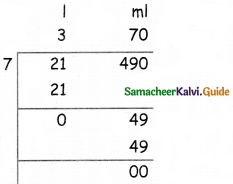21 l 490 ml ÷ 7 = 3 l 70 ml

(iv) 25 l 350 ml ÷ 5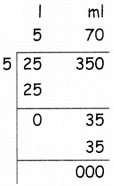25 l 350 ml ÷ 5 = 5 l 70 ml

Question 7.
Kalaiyarasi bought 5 l 500 ml groundnut oil and 750 ml sesame oil. How much oil did she bought in all?
Ground nut oil = 5 I 500 ml
Sesame oil = 750 ml
Total = 5 I 500 ml + 750 ml
= 6 l 250 ml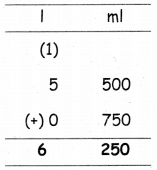Question 8.
In a fuel station there was 70 l 500 ml of fuel. How much amount of fuel will be left after selling 35 l 700 ml of fuel?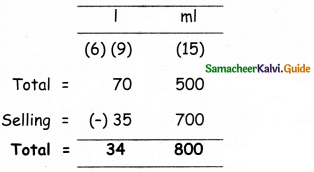Fuel left = 34 l 800 ml

Question 9.
A pot contains 9 l 500 ml of water, how much amount of water will 7 such pots contain?